## Creating Repeating Hyperbolic Patterns - Old and New

### Introduction

For more than a hundred years, mathematicians have drawn triangle tessellations in the Poincaré disk model of the hyperbolic plane, and some artists have found inspiration in these patterns. The Dutch graphic artist M. C. Escher (1898-1972) was most likely the first one to be so inspired. He used "classical" straightedge and compass constructions to create his hyperbolic patterns. More recently others have used computer graphics to create hyperbolic patterns. We will first explain how Escher drew the underlying tessellations for his patterns, then we will describe versions of the computer program that generated the design for the 2003 Math Awareness Month poster.

### Escher's Methods

In 1958, H. S. M. Coxeter sent Escher a copy of his paper Crystal Symmetry and its Generalizations [Coxeter1]. In his reply, Escher wrote:

"... some of the text-illustrations and especially Figure 7, page 11, gave me quite a shock." [Coxeter2]

Escher was shocked because that figure showed him the long-desired solution to his problem of designing repeating patterns in which the motifs become ever smaller toward a circular limit. Coxeter's Figure 7 contained the pattern of curvilinear triangles shown in Figure 1 below (but without the scaffolding). Of course that pattern can be interpreted as a triangle tessellation in the Poincaré disk model of the hyperbolic plane, although Escher was probably more interested in its pattern-making implications.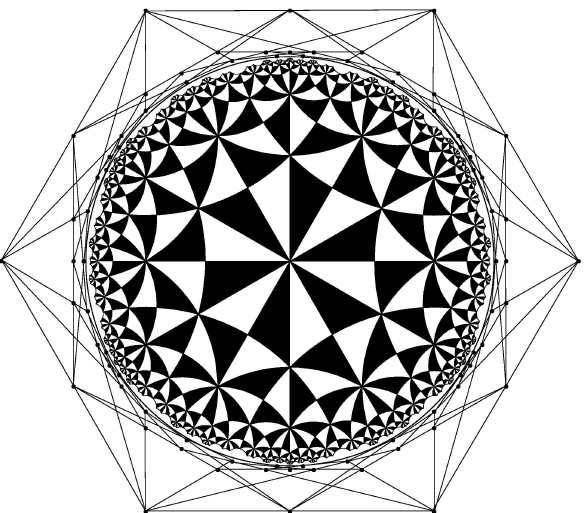Figure 1: The triangle tessellation of the Poincaré disk that inspired Escher, along with the "scaffolding" for creating it.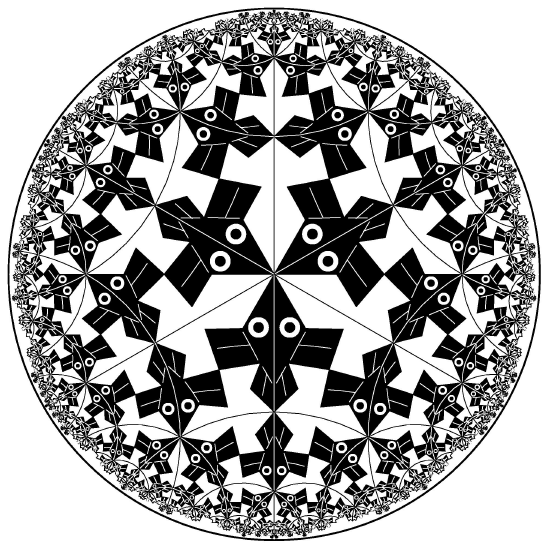Figure 2: A computer rendition of the design in Escher's print Circle Limit I.

The center of an orthogonal circular arc is external to the disk and is called its pole. The locus of all poles of arcs through a point in the disk is a line called the polar of that point [Goodman-Strauss]. The external dots in Figure 1 are the poles of the larger arcs, and the external line segments connecting them are parts of polars of the points of intersection of those arcs. The external web of poles and polar segments is sometimes called the scaffolding for the tessellation. The fact that the polars are lines can be used to speed up the straightedge and compass construction of triangle tessellations. For example, given two points in the disk, the center of the orthogonal arc through them is the intersection of their polars.

Coxeter explained the basics of these techniques in his return letter to Escher [Roosevelt], although by that time Escher had figured out most of this, as evidenced by Circle Limit I. Like Escher, mathematicians have traditionally drawn triangle tessellations in the Poincaré disk model using straightedge and compass techniques, occasionally showing the scaffolding. This technique was something of a geometric "folk art" until the recent paper by Chaim Goodman-Strauss [Goodman-Strauss], in which the construction methods were finally written down.

For positive integers p and q, with 1/p + 1/q < 1/2, there exist tessellations of the hyperbolic plane by right triangles with acute angles pi/p and pi/q. A regular p-sided polygon, or p-gon, can be formed from the 2p triangles about each p-fold rotation point in the tessellation. These p-gons form the regular tessellation {p,q} by p-sided polygons with q of them meeting at each vertex. Figure 3 below shows the tessellation {6,4} (with a central group of fish on top of it). As can be seen, Escher essentially used the {6,4} tessellation in Circle Limit I.

Goodman-Strauss constructs the tessellation {p,q} in two steps. The first step is to construct the central p-gon. To do this, he starts by constructing a regular Euclidean p-sided polygon P with center O that forms the outer edges of the scaffolding. Then he constructs the hyperbolic right triangle with a vertex angle of pi/p at O and its hypotenuse along a radius of P from O to one of the vertices A of P. The side of the right triangle through O is part of a perpendicular bisector of an edge of P containing A. The vertices of the entire central p-gon can then be constructed by successive (Euclidean) reflections across the radii and perpendicular bisectors of edges of P. The second step is to construct all the other p-gons of the tessellation. This could be done by first inverting all the vertices in the circular arcs that form the sides of the central p-gon, forming new p-gons, and then inverting vertices in the sides of the new p-gons iteratively as many times as desired. But Goodman-Strauss describes a more efficient alternative method using facts about the geometry of circles.

### Our First Method

In 1980 I decided to try to use computer graphics to recreate the designs in each of Escher's four Circle Limit prints (catalog numbers 429, 432, 434, and 436 in [Bool]). The main challenge seemed to be finding a replication algorithm that would draw each copy of a motif exactly once. There were two reasons for this. First, we were using pen-plotter technology then, so multiple redrawings of the same motif could tear through the paper. Efficiency was a second reason - the number of motifs increases exponentially from the center, and inefficient algorithms might produce an exponential number of duplications.

Moreover, I wanted the replication algorithm to build the pattern outward evenly in "layers", so that there would be no jagged edges. At that time, my colleague Joe Gallian had some undergraduate research students who were working on finding Hamiltonian paths in the Cayley graphs of finite groups [Gallian]. I thought that their techniques could also be applied to the infinite symmetry groups of Escher's Circle Limit designs. This turned out to be the case, although we found the desired paths in two steps.

The first step involved finding a Hamiltonian path in the Cayley graph of symmetry group of the tessellation {p,q}. This was done by David Witte, one of Gallian's research students. John Lindgren, a University of Minnesota Duluth student, implemented the computer algorithm, with me translating Witte's path into pseudo-FORTRAN [Dunham1].

The Cayley graph of a group G with a set of generators S is defined as follows: the vertices are just the elements of G, and there is an edge from x to y if y = sx for some s in S. Technically, this defines a directed graph, but in our constructions, the inverse of every element of S will also be in S, so for simplicity, we may assume that our Cayley graphs are undirected. As an example, the symmetry group of the tessellation {p,q} is denoted [p,q]. That symmetry group is the same as that of the tessellation by right triangles with angles pi/p and pi/q. The standard set of generators for the group [p,q] is {P,Q,R}, where P, Q, and R are reflections across the triangle sides opposite the angles pi/p, pi/q, and pi/2, respectively, in one such triangle.

There can be one-way or two-way Hamiltonian paths in the Cayley graphs of symmetry groups of hyperbolic patterns [Dunham3]. However, one-way paths are sufficient for our algorithms, so in this article "Hamiltonian path" will always denote a one-way Hamiltonian path.

There is a useful visual representation for the Cayley graphs of the groups [p,q], and thus for their Hamiltonian paths. A fundamental region for the tessellation {p,q} is a triangle that when acted on by the symmetry group [p,q] has that tessellation as its orbit. This fundamental region can be taken to be a right triangle lying on the horizontal diameter to the right of center, with its pi/p vertex at the center of the disk. This triangle is labeled by the identity of [p,q]. Each triangle of the tessellation is then labeled by the group element that transforms the fundamental region to that triangle. Thus each triangle represents a group element. To represent an edge in the Cayley graph, we draw a line segment connecting the centers of any two triangles sharing a side. Thus, there are three line segments out of each triangle, each representing the reflection across one side.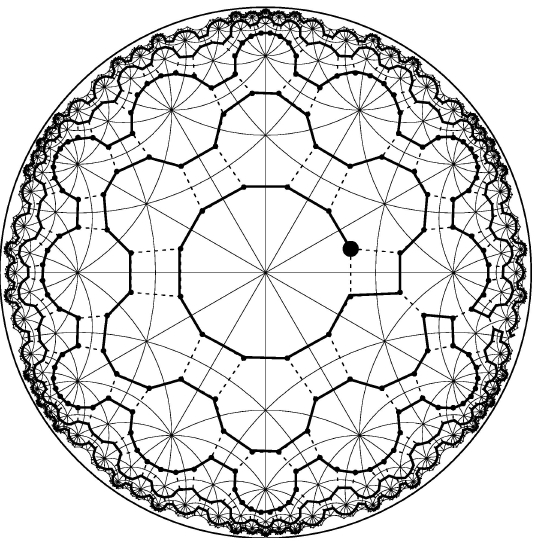Figure 3: The Cayley graph of the group [6,4], with a Hamiltonian path.

### Better Methods

A natural second step would have been to find Hamiltonian paths in the Cayley graphs of the symmetry groups of Escher's Circle Limit designs, which seemed possible since those symmetry groups were all subgroups of either [6,4] or [8,3]. However, we took a slightly bigger step instead, which also works for any symmetry group that is a subgroup of [p,q]. We first built up a super-motif (called the p-gon pattern in [Dunham1]) consisting of all the motifs adjacent to the center of the disk. The super-motif for the Circle Limit I design is shown in Figure 4, superimposed on top of the {6,4} tessellation.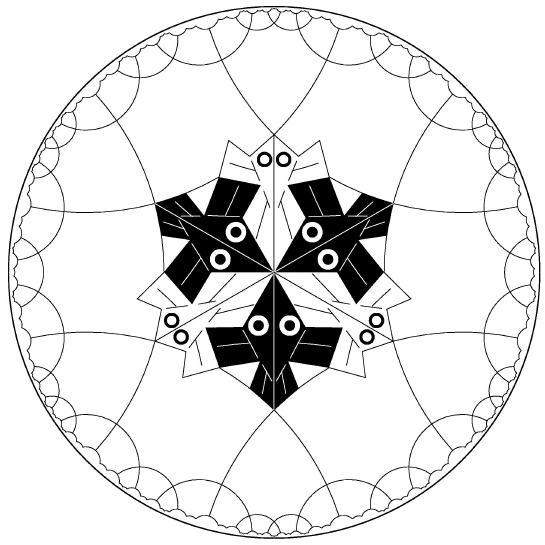Figure 4: The central "super-motif" for Circle Limit I.

The second step then consisted of finding Hamiltonian paths in "Cayley coset graphs". Conceptually, we form the cosets of a symmetry group by the stabilizer H of its super-motif. Then, by analogy with ordinary Cayley graphs, we define the vertices to be the cosets, and say that there is an edge from xH to yH if sxH = yH for some s in S.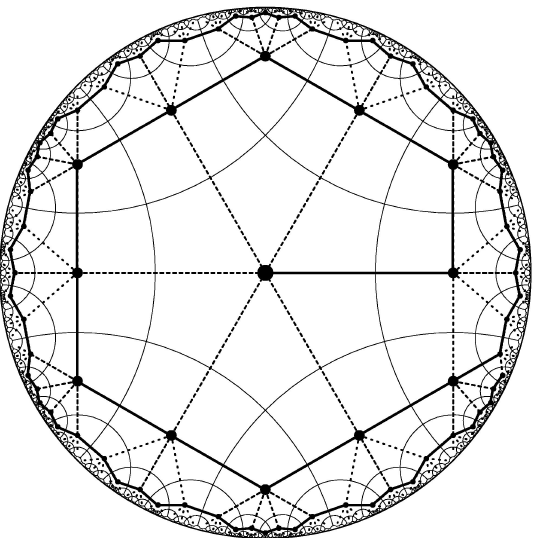Figure 5: A Hamiltonian path in a coset graph of [6,4].

One shortcoming of this method is that the Hamiltonian path must be stored in computer memory. This is not a serious problem, since the path can be encoded by small integers. That method essentially works by forming ever longer words in the generators from the edges of the Hamiltonian path. The transformations were represented by real matrices, which led to roundoff error after too many of them had been multiplied together to form the current transformation matrix. This was a more serious problem.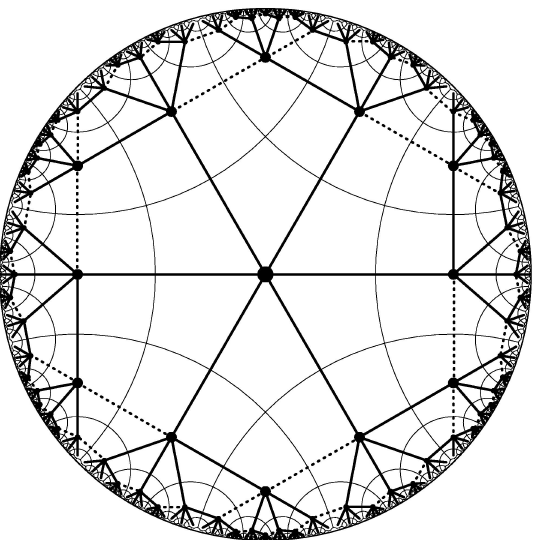Figure 6: A spanning tree in a coset graph of [6,4].

Other researchers have used different methods to generate a set of words in the generators of hyperbolic symmetry groups in order to replicate repeating hyperbolic designs. One method is to keep track of the transformations generated so far and iteratively add new transformations by multiplying all the previous transformations by the group generators, discarding duplicates. Then the desired pattern is generated by applying the final set of transformations to the motif. The techniques of automatic groups have been used to efficiently generate non-redundant sets of words. Silvio Levy has used this technique to create a computer rendition of Escher's Circle Limit III [Levy].

Like many mathematicians, I was immediately enthralled by M. C. Escher's intriguing designs when I first saw them, more than 30 years ago. Several years later when I became involved with computer graphics, that medium seemed like an obvious one to use to produce Escher-like designs. When discussing the symmetry groups of Escher's hyperbolic patterns with Joe Gallian, it occurred to me that Hamiltonian paths could be used as a basis for an algorithm to generate such patterns. At this point everything had come together and I could not resist the temptation to regenerate Escher's hyperbolic patterns with a graphics program. As described above, the students and I achieved this goal.

Having gone to the trouble of implementing a hyperbolic pattern program, I could not resist the further temptation of creating more hyperbolic patterns. I found inspiration in Escher's Euclidean repeating patterns. In constructing my patterns, I noticed myself paying attention to aesthetic issues such as shape and color. More of these hyperbolic designs can be seen in my chapter in the recent book Escher's Legacy [Schattschneider2]. When designing these patterns, I think of myself as working in the intersection of interesting mathematics, clever algorithms, and pleasing art.

### Acknowledgements

I would like to thank Doris Schattschneider for her considerable help, especially with the history of Escher and Coxeter's correspondence. I would also like to thank Abhijit Parsekar for help with the figures.

### References

[Coxeter1] Coxeter, H. S. M., "Crystal symmetry and its generalizations", Royal Society of Canada (3) 51 (1957), 1-13.

[Coxeter2] Coxeter, H. S. M., "The non-Euclidean symmetry of Escher's Picture `Circle Limit III'", Leonardo 12 (1979), 19-25, 32.

[Bool] Bool, F.H., Kist, J.R., Locher, J.L., and Wierda, F., editors, M. C. Escher, His life and Complete Graphic Work, Harry N. Abrahms, Inc., New York, 1982. ISBN 0-8109-0858-1

[Dunham1] Dunham, D., J. Lindgren, and D. Witte, "Creating repeating hyperbolic patterns", Computer Graphics, 15 (1981), no. 3, 79-85.

[Dunham2] Dunham, D., "Hyperbolic symmetry", Computers and Mathematics with Applications, Part B 12 (1986), no. 1-2, 139-153.

[Dunham3] Dunham, D., D. Jungreis, D. Witte, Infinite Hamiltonian paths in Cayley digraphs of hyperbolic symmetry groups, Discrete Mathematics, 143, 1995, 1-30.

[Goodman-Strauss] Goodman-Strauss, Chaim, "Compass and straightedge in the Poincaré disk", Amer. Math. Monthly, 108 (2001), no. 1, 38-49.

[Greenberg] Greenberg, Marvin, Euclidean and Non-Euclidean Geometries, 3rd Edition, W. H. Freeman and Co., 1993. ISBN 0-7167-2446-4

[Levy] Levy, Silvio,. Escher Fish, at: http://geom.math.uiuc.edu/graphics/pix/Special_Topics/Hyperbolic_Geometry/escher.html

[Roosevelt] The letter of December 29, 1958 from H. S. M. Coxeter to M. C. Escher, from the Roosevelt collection of Escher's works at The National Gallery of Art, Washington, D. C.

[Schattschneider1] Schattschneider, Doris, A picture of M. C. Escher's marked reprint of Coxeter's paper , private communication.

[Schattschneider2] Schattschneider, Doris, and Michele Emmer, editors, M. C. Escher's Legacy: A Centennial Celebration, Springer Verlag, 2003. ISBN 3-540-42458-X

Page URL: http://www.d.umn.edu /~ddunham/notices/paper.html
Page Author: Doug Dunham
Last Modified: Thursday, 06-Feb-2003 15:53:10 CST
Comments to: ddunham@d.umn.edu